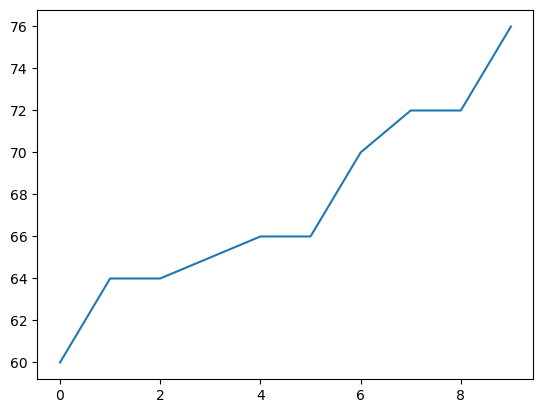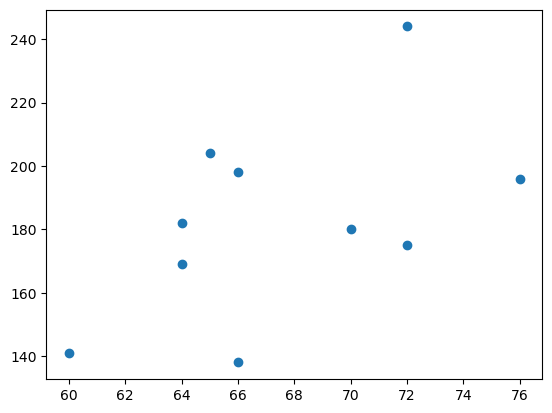# How to create basic plots

## Description

Plotting is a huge topic with many options and variations, but the most foundational types of plots are a line plot and a scatterplot. How can we create those?

Related topics:

## Using Matplotlib, in Python

View this solution alone.

We will create some fake data using Python lists, for simplicity. But everything we show below works also if your data is in columns of a DataFrame, such as df['age'].

1
2
3
patient_id     = [   0,   1,   2,   3,   4,   5,   6,   7,   8,   9 ]
patient_height = [  60,  64,  64,  65,  66,  66,  70,  72,  72,  76 ]
patient_weight = [ 141, 182, 169, 204, 138, 198, 180, 175, 244, 196 ]


The conventional way to import matplotlib in Python is as follows.

1
import matplotlib.pyplot as plt


Make a line plot by giving the $x$ and $y$​ data values in separate lists (or in this case pandas Series). This line plot is very jagged just because the data was random.

1
2
plt.plot( patient_id, patient_height )         # create plot
plt.show()                                     # display plotYou can make a scatterplot as follows.

1
2
plt.scatter( patient_height, patient_weight )  # create plot
plt.show()                                     # display plotIf your data is already in a pandas DataFrame, there are shortcuts:

1
2
3
4
5
6
7
8
9
10
11
12
13
14
15
# Plot all columns:
df.plot()
plt.show()

# Plot all columns in separate subplots:
df.plot( subplots = True )
plt.show()

# Plot one column:
df['column'].plot()
plt.show()

# Plot specific columns:
df.plot( x='col name', y='other col name' )
plt.show()


See a problem? Tell us or edit the source.

## Solution, in R

View this solution alone.

We will create some fake data using vectors, for simplicity. But everything we show below works also if your data is in columns of a DataFrame.

1
2
3
patient.id     <- c(0, 1, 2, 3, 4, 5, 6, 7, 8, 9)
patient.height <- c(60,  64,  64,  65,  66,  66,  70,  72,  72,  76)
patient.weight <- c(141, 182, 169, 204, 138, 198, 180, 175, 244, 196)


We can make a line plot if we use the type="l" option (which is an “ell,” not a number one).

1
plot(patient.id, patient.height, main="Patient heights", type="l")


We can create a scatterplot if we have two numerical columns, such as the height and weight in the data above.

1
plot(patient.height, patient.weight, main = "Height vs. Weight")